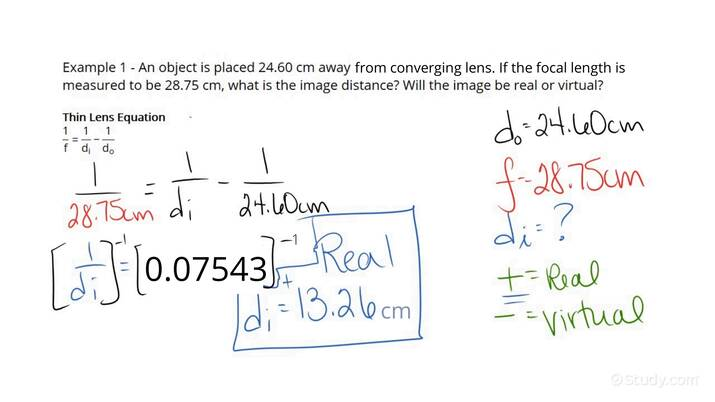# Determining if an Image is Real or VirtualAn error occurred trying to load this video.

Try refreshing the page, or contact customer support.

• 00:04 Determining if an…
• 02:28 Determining if an…
APRIL MANGANO, Kirsten Wordeman
• Instructors
APRIL MANGANO

April has been tutoring students, elementary to college level, in varying subjects for over 10 years. April has a Bachelor of Physics from Rutgers University and is currently working toward a Master's of Applied Physics from John's Hopkins University.

•
Kirsten Wordeman

Kirsten has taught high school biology, chemistry, physics, and genetics/biotechnology for three years. She has a Bachelor's in Biochemistry from The University of Mount Union and a Master's in Biochemistry from The Ohio State University. She holds teaching certificates in biology and chemistry.

## Determining if an Image is Real or Virtual

Step 2: Take the values given within the problem and insert them into the Thin Lens equation to determine if an image is real or virtual.

## What are Real and Virtual Images and What is their Relationship

Real Image: It is an image that forms from converging light rays and are created when objects are located outside the focal length of the converging lenses. Some of their characteristics included that they always appear inverted, can be seen on a screen, and are formed from both concave and convex lenses. In comparison, a virtual image is an image that forms.

Virtual Image: It is an image that forms when light rays diverge and are created when objects are located inside the focal length of the converging lenses. Some of their characteristics include that they always appear upright, cannot be seen on a screen, and that the light rays creating them will never intersect.

Thin Lens Equation: To determine the position of an image this equation can be used. The equation is as follows

{eq}\frac{1}{f}= \frac{1}{d_i} + \frac{1}{d_o} {/eq}

Where f equals the focal length, {eq}d_i {/eq} equals the image distance, and {eq}d_o {/eq} equals the object distance.

Understanding the Thin Lens Equation: Positive focal length will indicate a convex mirror and converging lenses. The negative focal length will indicate a concave mirror and diverging lenses. Positive image distance will indicate a real image and a negative image distance will indicate a virtual image.

The following two examples will illustrate how to determine if an image is real or virtual by using the Thin Lens equation.

## Examples of Determining if an Image is Real or Virtual

### Example 1

If an object is placed 15 cm in front of a diverging lens that has a focal length of - 8.2cm, what will the image distance be? Will the image be real or virtual?

After reading the problem, we know that we must use the information seen within the problem and insert the values into the Thin Lens equation

Step 2: Take the values given within the problem and insert them into the Thin Lens equation to determine if an image is real or virtual

After inserting values into the equation, we will get the following

{eq}\frac{1}{-8.2cm}= \frac{1}{d_i} + \frac{1}{15cm} {/eq}

Now solving for the image distance, we will get the following,

{eq}\frac{1}{-8.2cm}= \frac{1}{d_i} + \frac{1}{15cm} {/eq} where {eq}d_i {/eq} equals -5.3 cm. The image distance is negative, therefore, the image is virtual.

### Example 2

If an object is placed 22 m in front of a converging lens that has a focal length of 8 m, what will the image distance be? Will the image be real or virtual?

After reading the problem, we know that we must use the information seen within the problem and insert the values into the Thin Lens equation

Step 2: Take the values given within the problem and insert them into the Thin Lens equation to determine if an image is real or virtual

After inserting the values into the equation, we will get the following

{eq}\frac{1}{8m}= \frac{1}{d_i} + \frac{1}{22m} {/eq}

Now solving for the image distance, we will get the following,

{eq}\frac{1}{8m}= \frac{1}{d_i} + \frac{1}{22m} {/eq} where {eq}d_i {/eq} equals 12.57 m. The image distance is positive, therefore, the image is real.